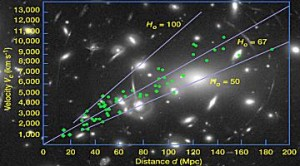It was the Astronomer Edwin Hubble that was able to describe in numerical terms at which the rate of the universe is expanding, this is known as the Hubble’s Law. In 1929, he was able to observe that almost all galaxies seemed to be moving away from the Earth. This is illustrated in mathematical terms as:

H= v/d

Where H is the Hubble’s constant, v refers to galaxy’s radial outward velocity or speed and d is the distance from the Earth. Based on this relationship, the farther the galaxy, the faster it is moving away from Earth. It is not easy to determine the exact value of Hubble constant. This is because the two quantities required are difficult to measure especially the exact distance of a galaxy from the Earth. The current value of this constant as calculated by Edwin Hubble as 500 km/s/Mpc or 500 kilometer per second per megaparsec.

Hubble’s Law is very important in astronomy and cosmology. It was the first concrete support for Einstein’s theory of Relativity being applied to the universe. The determined value of Hubble’s constant can be used to estimate an age of the universe. It also helps validate theories of Dark matter and Dark energy. A recent discovery in 1998 revealed that the expansion of the universe is accelerating.

Hubbles Law